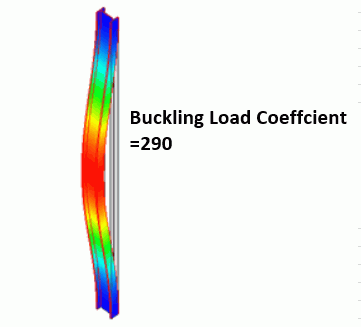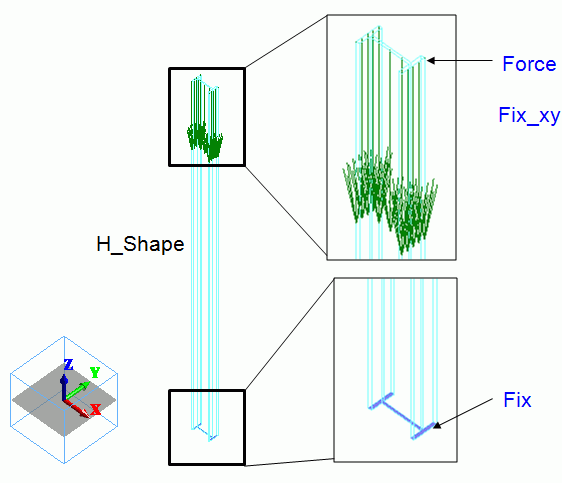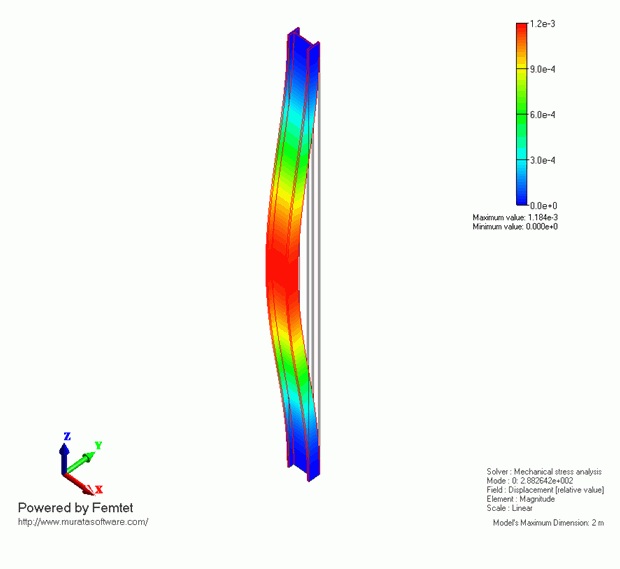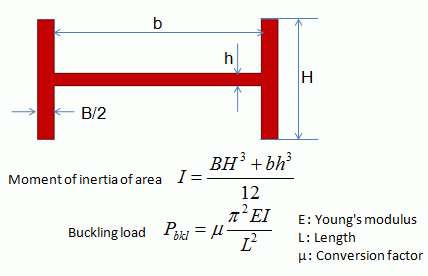﻿ Buckling AnalysisExamples | Product | Murata Software Co., Ltd.Example48Buckling AnalysisGeneral

• The buckling analysis is performed for an H-beam steel.

• The object’s buckling load factor and the buckling modes are solved.

• Unless specified in the list below, the default conditions will be applied.

Analysis Space

 Item Settings Analysis Space 3D Model unit mm

Analysis Conditions

The analysis type is buckling analysis

 Item Settings Solvers Mechanical Stress Analysis [Galileo] Analysis Type Buckling analysis

Model

An H-shaped sheet body is created on XY plane. It is extruded to form an H-shape pillar.
The bottom face is fixed. The top face is loaded downward and fixed in X and Y directions. Uniform displacement is set on it.*

* The uniform displacement prevents the rotational displacement.Body Attributes and Materials

 Body Number/Type Body Attribute Name Material Name 0/Solid H_shape 104_Stainless_steel *

* Available from the Material DB

Boundary Conditions

 Boundary Condition Name/Topology Tab Boundary Condition Type Settings Fix/Face Mechanical Displacement Select all X/Y/Z components. UX=0, UY=0, UZ=0 Force/Face Mechanical Load Select “Set the total load”. X=0.0, Y=0.0, Z=-1000 Fix_xy/Face Mechanical Displacement Select X and Y components. UX=0, UY=0 Select “Uniform displacement” Select UX, UY and UZ.

Results

The following will be output on the Output Window or the log file.

 <> Buckling load factor Mode[ 0] = 2.88264161e+002   Multiply the original load with the buckling load factor to acquire the buckling load. Field values such as displacement, strain, stress, etc acquired by the buckling analysis are all relative.

In this model, the buckling load factor is 288.

The load set on “Force” is 1000[N]. Therefore, the buckling load is 288[KN]

The displace diagram is shown below. The contour diagram shows the displacement. The displacement is relative.Compare with Theoretical Values

The buckling load for a H-shape pillar is given by the following formula.In this case,

B/2 = 7e-3[m]

b = 86e-3[m]

H = 50e-3[m]

h = 5e-3[m]

E = 2e11[Pa]

L = 2.0[m]

μ＝4, which means that there is no horizontal or rotational displacement for either end.

These values yield I = 14.7e-8[m4] and Pbkl = 290[KN]

The result of Femtet matches this Pbkl quite well.

﻿